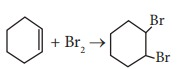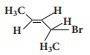# Chemistry - Online Test

Q1. Select the molecule which has only one π bond.
Explaination / Solution:
No Explaination.

Q2.

For the following reactions

(A) CH3CH2CH2Br + KOH CH2 = CH2 + KBr +H2O

(B) (CH3)3CBr + KOH (CH3)3 COH + KBr

(C)Which of the following statement is correct?

Explaination / Solution:
No Explaination.

Q3. 40 ml of methane is completely burnt using 80 ml of oxygen at room temperature The volume of gas left after cooling to room temperature is
Explaination / Solution:

CH4(g) + 2O2(g) → CO2 (g)+ 2 H2O (l)Since the product was cooled to room temperature, water exists mostly as liquid. Hence, option (a) is correct

Q4. The correct statement regarding the comparison of staggered and eclipsed conformations of ethane, is
Explaination / Solution:
No Explaination.

Q5. Electronic configuration of species M2+ is 1s2 2s2 2p6 3s2 3p6 3d6 and its atomic weight is 56. The number of neutrons in the nucleus of species M is
Explaination / Solution:

M2+ : 1s2 2s2 2p6 3s2 3p6 3d6

M : 1s2 2s2 2p6 3s2 3p6 3d8

Atomic number = 26

Mass number =56

No. of neutrons = 56 -26 = 30

Q6. The IUPAC name ofis
Explaination / Solution:
No Explaination.

Q7. What would be the IUPAC name for an element with atomic number 222?
Explaination / Solution:
No Explaination.

Q8. The gaseous envelope around the earth is known as atmosphere. The region lying between an altitudes of 11-50 km is __________
Explaination / Solution:
No Explaination.

Q9. Which of the following statements about hydrogen is incorrect ?
Explaination / Solution:

Correct statement : Hydrogen has three isotopes of which protium is the most common.

Q10. For alkali metals, which one of the following trends is incorrect ?
Explaination / Solution:

Potassium is lighter than sodium (Refer table 5.3)

The correct order of density is Li < K < Na < Rb < Cs

0.54 < 0.86 < 0.97< 1.53< 1.90(in g cm–3)